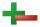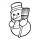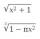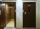1. MistakeNicol mistake when calculate in school. Instead of add number 20 subtract it. What is the difference between the result and the right result?
2. Opposite numbersCalculate opposite numbers (additive inverse) to given ones:
3. ThermometerThe thermometer showed -3°C at morning. Then temperature was increased by 1°C again increased by 1°C and then decreased by 1°C and then decreased by 4°C. Which terminal temperature thermometer shows?
4. With bracketCalculate (evaluate) simple mathematical expression with a negative numbers and a bracket: 13+15*5-2*(-6)
5. ElevatorThe panel house has ten over-ground floors and four underground. The lift goes from the ground floor to 2nd floors, then goes down to 3rd underground floors, then 9 floors up and finally 4 floors down. To what story elevator come? How many floors did it cr
6. Thermometerthermometer showed -12 degrees Celsius at the morning then the temperature rises by 4 degrees and later again increased by 2 degrees at the evening has fallen by 5 degrees and then fall 3 degrees. What end temperature does the thermometer show?
7. Expression 6Evaluate expression: -6-2(4-8)-9
8. Brackets 2Add parenthesis to make true: 5-2×6-4+2=5
9. Expression plus minusEvaluate expression: (-1)2 . 12 – 6 : 3 + (-3) . (-2) + 22 – (-3) . 2
10. The temperatureThe temperature at 1:00 was 10 F. Between 1:00 and 2:00, the temperature dropped 15F. Between 2:00 and 3:00, the temperature rose 3F. What is the temperature at 3:00?
11. Evaluate expression 2Evaluate expression with negatives: (-3)+4+(-8)+(-6)+4+(-1)

We apologize, but in this category are not a lot of examples.
Do you have an interesting mathematical example that you can't solve it? Enter it, and we can try to solve it.

To this e-mail address, we will reply solution; solved examples are also published here. Please enter e-mail correctly and check whether you don't have a full mailbox.